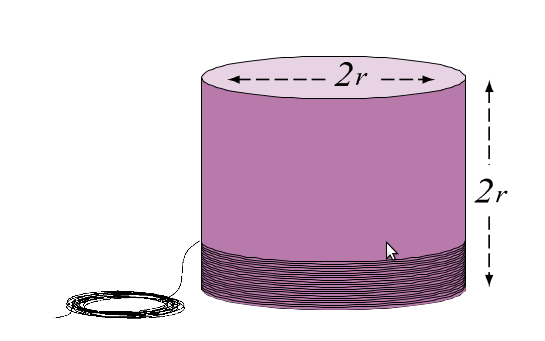Surface area of a sphere

## Objective:

To give a suggestive demonstration of the formula for the surface area of a sphere in terms of its radius.

## Related terms

### Sphere:A sphere is a perfectly round geometrical and circular object in three-dimensional space that resembles the shape of a completely round ball. Like a circle, which, in geometrical contexts, is in two dimensions, a sphere is the set of points that are all the same distance r from a given point in space. This distance r is the radius of the sphere, and the given point is the center of the sphere. The maximum straight distance through the sphere passes through the center and is thus twice the radius; it is the diameter.

## Surface Area:The surface area of a sphere is: A = 4 π r2.

The cylinder is twice the length needed to cover the hemisphere. Since the thickness of the thread is uniform and the same for both the threads,surface areas are proportional to the lengths of the threads approximately.